# How to count non-empty cells in Excel

This articles looks at the ways to count non-blank cells in Excel 365 - 2013. Below you'll find 3 methods for counting non-blanks: see the number on the Excel Status bar, employ the Find and Replace dialog or use a special formula.

Your table may have numerous blank cells left for better visualization. On the one hand, such layout is really convenient. On the other hand, it may prevent you from seeing the correct number of data rows. e.g. how many products are sold or how many people take part in a conference.

If you aim to count empty cells, you'll find a few quick ways in the above-linked article.

Below there are 3 options for counting non-blank cells in Excel.

Note. If a cell contains a formula that returns space between quotes (""), it's not seen as empty. I will refer to them as to blank formulas in this article.

## Count option on the Excel Status bar

Excel Status bar shows a number of tools you may find helpful. Here you can see page layouts, zoom slider and basic math functions displayed for numerical values.

To see how many selected cells contain data, just look at the COUNT option on the Status bar.

Note. This option will not work if you have only one filled cell in the selected range.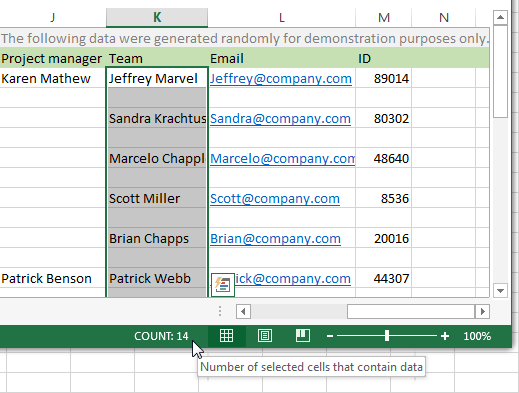## Excel - count non-blank cells with the Find and Replace option

It's also possible to count non-empty cells with the help of the standard Excel Find and Replace dialog. This method is good if you have a large table. You'll get all values displayed on one window along with their cell addresses. In addition, you can easily navigate to any item by clicking on its name in the list.

1. Select the range where you need to count the non-blanks and press the Ctrl + F hotkey.
2. You will see the Find and Replace dialog box. Enter the asterisk symbol ( * ) in the Find what field.3. Press the Options button and pick the Values or Formulas item from the Look in: drop-down list.
• If you select Values, the tool will count all filled cells and ignore blank formulas.
• When you pick Formulas, Find and Replace shows all cells that have values and any formulas.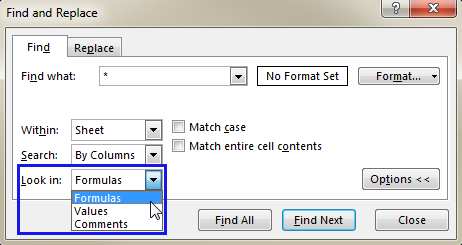4. Click Find All to see the results. You'll get all the found items and their quantity on the pane.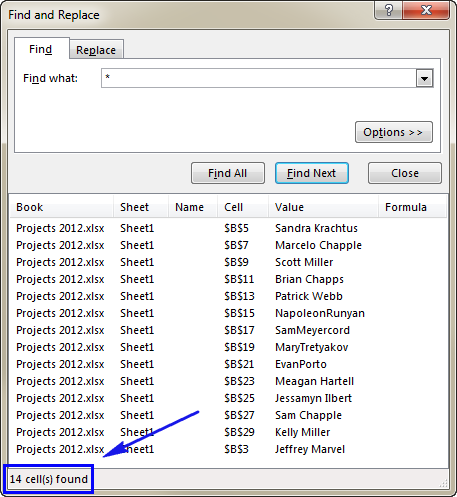Tip. You can now select all found items on the Find and Replace pane. You'll see all non-blank cells highlighted and it will stay after you close the window.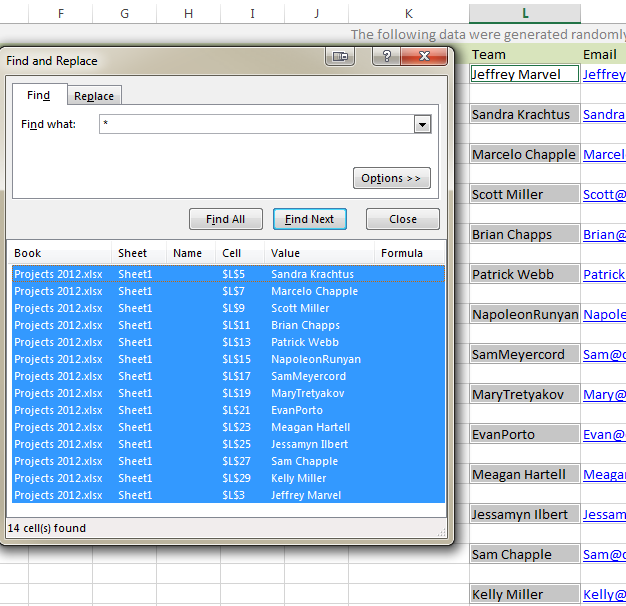## Use a special Excel formula to count all non-blank cells

The third way to calculate the number of non-empty cells is to use an Excel formula. Though you will not see where the cells are, this option helps you choose what kinds of filled cells you want to count.

If you need to count all filled cells, constants, formulas, cells with spaces, you should use the formula `=COUNTA()`.

To get the number of cells with constants and those including spaces, enter

`=ROWS(L8:L11) * COLUMNS(L8:L11)-COUNTBLANK(L8:L11)`

Follow these steps to apply the formulas:

1. Select any empty cell in your sheet.
2. Enter `=counta()` or `=ROWS() * COLUMNS()-COUNTBLANK()` to the formula bar.
3. Then you can manually enter the range address between the brackets in your formula. Or place the mouse cursor between the brackets and highlight the necessary cell range in your table. You will see the address automatically appear in the formula.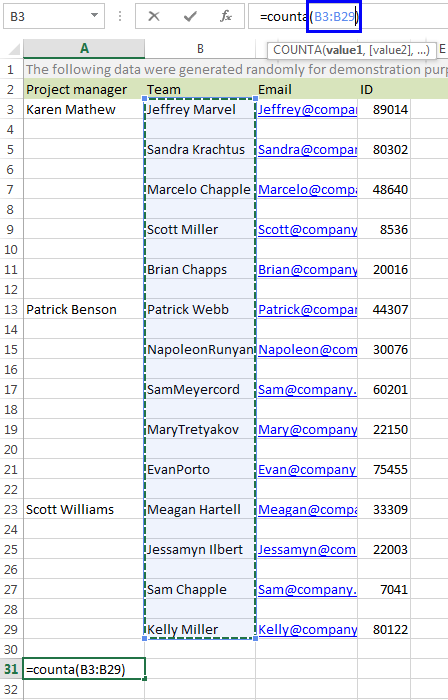With the formula `=ROWS() * COLUMNS()-COUNTBLANK()` you need to enter the range address 3 times.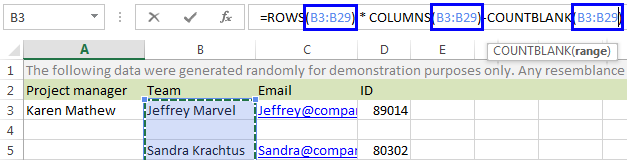4. Press Enter on your keyboard.

You will see the result in the selected cell.

If you want to count only constants without cells with extra spaces, use `=SUM(--(LEN(TRIM(range))>0))` Please note, this is an array formula that needs to be entered with CTR + Shift + Enter.

1. Select any empty cell in your sheet.
2. Enter `=SUM(--(LEN(TRIM())>0))` in the formula bar.
3. Place your mouse cursor between the brackets and select the range in your table. You will see the range address appear in the formula.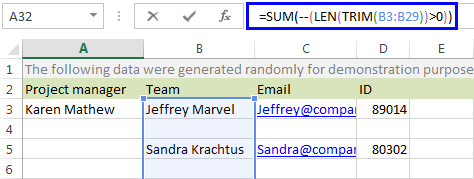4. Press Ctrl + Shift + Enter to see the number in the selected cell.

On the screenshot below, you can see a brief summary showing how these 3 formulas work with constants, blank formulas and extra spaces. In the test table I have a range with 4 cells selected. A2 contains a value, A3 has a formula that returns an empty string, A4 is empty and A5 has two spaces entered. Under the range, you can see the number of the found cells next to the formula I used to find them.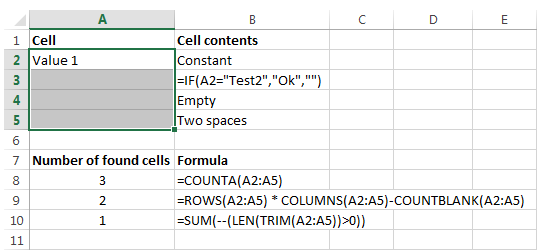Another way to count non-blanks in Excel is using the COUNTIF formula `=COUNTIF(range,"<>"&"")`. You will find the full details in this tutorial - COUNTIF for non-blanks.

Now three ways of counting non-blank cells in Excel are at your disposal. Just pick the one that suites you best. It can be the Status bar, Find and Replace or a formula. Be happy and excel in Excel!

## You may also be interested in

1. I try this and it works correctly

value = Worksheets("Sheet1").Range("A:A").Cells.SpecialCells(xlCellTypeConstants).Count

Or if you are in the current sheet just:

value = Range("A:A").Cells.SpecialCells(xlCellTypeConstants).Count

2. I disagree with Imran. Although posted a while ago this remains a really nice tutorial with good examples illustrating how to handle spaces and blank cells with various Excel formulae.

3. Hello, Maria Azbel, It will be more comfortable if you describe properly how this formula works...

4. A really great explanation thanks.
It does a wonderful job of detailing the difference between a formula that returns a blank and an empty cell.

The next level of refinement would be to have a formula that counts non blank visible cells.

I have a table that I use drop down filters on.
I only want to count the visible non blank cells.
Do you have any suggestions please?

Subtotal and Aggregate functions are the first obvious choice, but they get confused with formulas that return blanks.